Community /

# Tagging stimulus events using OpenBCI: Part 1

In brain research, timing is very important. Brain responses to stimuli (like a picture on a computer screen) are very fast and very dynamic, changing rapidly during the different steps of stimulus processing. In order to have a clear picture of the dynamics of brain activity, you have to be able to accurately tag when the events are occuring relative to the brain activity that you are recording. This is a difficult problem for which there are many possible solutions. In this experiment/tutorial/exploration, I will step through our first attempt to get a handle on tagging brain responses to external events using the OpenBCI hardware and some stimulus presentation software we are developing called OpenEXP and the openbci-sdk, an npm module to interact and stream data from the openbci hardware written in javascript.

The preliminary approach we are taking here is to send a value from the OpenEXP stimulus presentation software everytime a stimulus (here, a picture) is presented to the computer screen over the wireless connection to the OpenBCI board, and embed that value in one of the aux channels on the openbci board so that it can be used to tag the presentation of a picture with the brain response. While this solution is relatively simple to implement (gulp), there are a few concerns right off the bat:

• There is an unknown delay between when the software sends the trigger (value) and when it is embedded into the aux channel on the board
• There could be variability in the time that the stimulus is presented due to the refresh rate of the computer
• There are almost certainly other sources of variance that I have not yet though about.

Why is the timing so important?? Well here’s a little simulation to illustrate why. Imagine that you have an experiment where you are presented a series of pictures and recording the brain activity linked to those pictures. Here is a simulated brain response to a picture:

``` # let's first import some useful libraries import pandas as pd import numpy as np import matplotlib.pyplot as plt import seaborn as sns import scipy as sp %matplotlib inline ```

``` Fs = 250 f = 5 sample = 1000 x = np.arange(sample) y = np.sin(2 * np.pi * f * x / Fs) y[0:sample/2]=0 y[sample/2+50:]=0 plt.plot(x, y) plt.xlabel('sample') plt.ylabel('voltage') plt.show() ```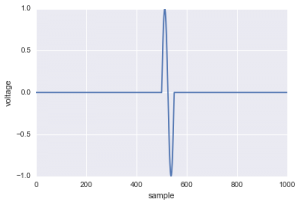This is certainly overly simplified, but works for this demonstration. In a typical experiment, you may record the brain response usually to hundreds of events, and then average over those events to reduce some of the noise that is unavoidable in brain research. Let’s add in a little noise to make it a little more realistic:

``` y_plus_noise = [i + np.random.randn(1)*.3 for i in y] plt.plot(x, y_plus_noise) plt.xlabel('sample') plt.ylabel('voltage') plt.show() ```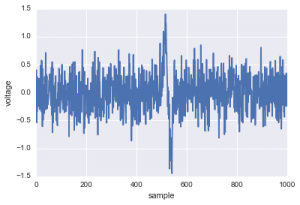There, that’s a little more like it. In fact, I would be excited if I recorded brain data and it looked like that. So, in a typical brain science experiment, you might average over a number of trials to reduce some of the this noise. Let’s see what happens when we average over some of these simulated trials: Let’s see how the number of trials influences our final estimate of the brain activity. We’ll use 10, 50 and 100 trials:

``` num_trials = 10 trials = [];```

```for trial in range(num_trials): trials.append([i + np.random.randn(1)*.3 for i in y]) mean_trials = np.mean(trials,0) plt.plot(x, mean_trials) plt.title('Average over 10 trials') plt.xlabel('sample') plt.ylabel('voltage') plt.show()```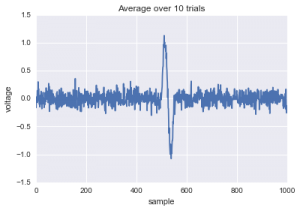```num_trials = 50 trials = []```
``` for trial in range(num_trials): trials.append([i + np.random.randn(1)*.3 for i in y]) mean_trials = np.mean(trials,0) plt.plot(x, mean_trials) plt.title('Average over 50 trials') plt.xlabel('sample') plt.ylabel('voltage') plt.show()```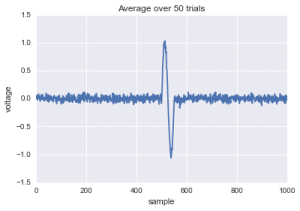```num_trials = 100 trials = []```

```for trial in range(num_trials): trials.append([i + np.random.randn(1)*.3 for i in y]) mean_trials = np.mean(trials,0) plt.plot(x, mean_trials) plt.title('Average over 100 trials') plt.xlabel('sample') plt.ylabel('voltage') plt.show()```Look at that! Even when there is random noise in the data, you can average over trials to recover the ‘real’ brain signal. What about when there is *ALOT* of noise in the data? Let’s test it.

``` y_plus_noise = [i + np.random.randn(1) for i in y] plt.plot(x, y_plus_noise) plt.title('One very noisy trial') plt.xlabel('sample') plt.ylabel('voltage') plt.show()```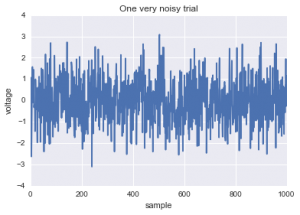```num_trials = 100 trials = []```

```for trial in range(num_trials): trials.append([i + np.random.randn(1) for i in y])```

```mean_trials = np.mean(trials,0) plt.plot(x, mean_trials) plt.title('Average over 100 very noise trials') plt.xlabel('sample') plt.ylabel('voltage') plt.show()```Yep, you can still see that brain response, even if it’s not visibly there on a single trial. It looks like averaging over 100 very noisy trials is analogous to averaging over 10 very clean trials.

Ok, now that we know that we can use averaging to remove noise from the signal, what happens if there is some variability in *when* the stimulus is tagged? For example, if we send a event tag (i.e. a *trigger*) from our software, what happens if there is some variable delay in when it actually gets to the hardware? Let’s simulate it:

``` y_plus_noise = [i + np.random.randn(1)*.3 for i in y] plt.plot(x, y_plus_noise) plt.title('One trial') plt.xlabel('sample') plt.ylabel('voltage') plt.show()```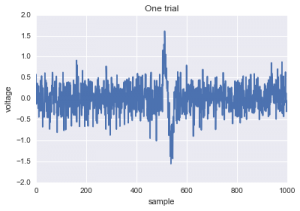```y_plus_noise = np.roll([i + np.random.randn(1)*.3 for i in y],-200) plt.plot(x, y_plus_noise) plt.title('One trial - shifted in time') plt.xlabel('sample') plt.ylabel('voltage') plt.show()```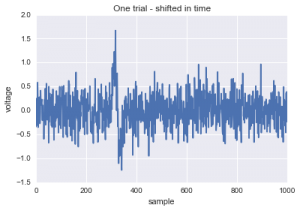```num_trials = 100 trials=[]```

```for trial in range(num_trials): shiftAmount = np.random.randint(-50,50,1) trials.append(np.roll([i + np.random.randn(1)*.3 for i in y],shiftAmount)) ```
```mean_trials = np.mean(trials,0) plt.plot(x, mean_trials) plt.title('Average over 100 trials jittered in time') plt.xlabel('sample') plt.ylabel('voltage') plt.show()```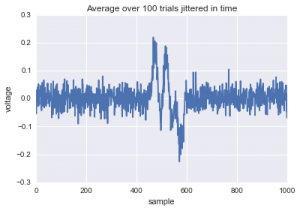Ouch, that really screws up the averaging. Not only does the waveform look funky, it also reduces the voltage range quite a bit. That’s not good. This is why timing is so important. We could go further here and quantify how the variance in the latency of the trigger changes the average brain response, but for the sake of simplicity, let’s just say that latency is bad and we want to minimize it, or at least make it very consistent so that we can correct for it.

# Chapter 2: Empirically testing the trigger delay

Now that we’ve established that a variable latency is bad, let’s empirically test the latency in our specific system. How will we do this, you ask? In order to measure the delta (difference) between when the stimulus is actually presented to the screen and when it is tagged in the data, we first have to have a tag of when the picture was shown on the screen. Before going into detail, here is the gist of how we solved this problem. First, we designed an experiment where we present 100 pictures (specifically, black circles) to the computer screen at a rate of about 1 second per trial, with 1 second between each presentation. Then, we taped a photoresistor to the computer screen to measure the precise moment that the picture came on the screen. We wired the photoresistor into the openbci board and output the data on one of the aux channels (for more on this, go to this post, coming soon). Can you see where we are going with this? Finally, to measure the latency, we simply computed the temporal difference between when the picture was actually presented to the screen (from the photoresistor) and when the software trigger made it to the openbci board over the wireless connection. TADA!

``` # read in the data data = pd.read_csv('/Users/andrewheusser/Dropbox/openbci/triggerTests/triggerTest.txt',names=['software','hardware'])```

```# a snippet of the data plt.plot(data[:15000]) plt.title('Hardware and software trigger data') plt.xlabel('samples (250hz)') plt.ylabel('value (arbitrary)') plt.show()```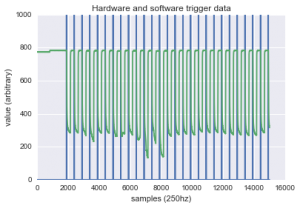```# zoom in plt.plot(data[5200:6000]) plt.title('Zooming in..') plt.show()```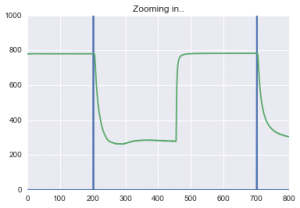```# zoom in more plt.plot(data[5900:5915]) plt.title('Even closer..') plt.show()```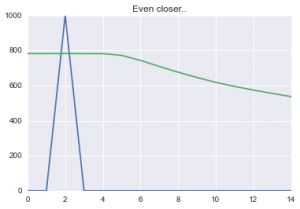Ok so what are we looking at here? The blue line represents the software trigger, and the green line represents the hardware trigger. Each time the stimulus is tagged in from the software, a value of 1000 is inserted into the data stream. Each time the photoresistor detects a change on the screen, the values go from higher to low. This is because we are presenting a black stimulus on a white background. If we had presented a white stimulus on a black background, this pattern would be reversed (i.e. starting low and going high when the picture is presented).

So, what do we notice about this pattern. First, the two events consistently occur within a few samples of each other (1 sample every 4ms). Great! That’s excellent news. Second thing to notice is the difference in the shape of the two time courses. The software trigger is a binary value, either it was presented or it wasn’t. Thus, we see a single peak each time it occurs. The photoresistor, on the other hand, displays a more organic waveform. As the photoresistor transitions from high light (white background) to low light (black stimulus) there is a gradual fall off. Uh oh, this isn’t really what we are looking for. We want to know the precise moment that the picture was presented. You’ll notice that the photoresistor remains steady at ~800 and then drops quickly, but smoothly. To deal with this, see decided to normalize both lines, and then set a threshold on the photoresistor data. Essentially, we specified that any time the normalized values fell below a threshold of .925, we quantified that as the moment in time (sample in time) when the picture was presented. The red line in the figure below shows this plot.

Finally, note that the blue line (software) actually occurs before the green line (photoresistor). Wait, what? How is it that the software tag occurs before the physical event has occured? Can this be explained by time travel or quantum mechanics? Maybe, but the most parsimonious explanation is that refresh rate of the computer has something to do with this, and may also be due to the order in which the software evaluates the code. Stay tuned for a Part II as we actively investigate what is going on here. In the meantime, let’s push forward and quantify our delay.

``` # z-score hardware trigger data data['hardware'] = (data['hardware'] - data['hardware'].mean()) / data['hardware'].std()```

```# define a threshold data['hardware_bool'] = (data['hardware']<.925).astype('int') # binarize the software trigger data data['software'] = (data['software']>1).astype('int') software_bool = data.index[data['software']==1]```

```# plot to show all three points plt.plot(data[5400:5410]) plt.xlabel('sample') plt.ylabel('normalized value') plt.show()```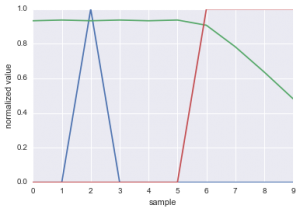In the figure above you can see that the red line jumps from 0 to 1 the moment that the green line falls below .925, indicating that the stimulus was presented. What we are ultimately interested in is the difference between the blue peak and the red peak

We computed this delta value for ~80 stimulus presentations and plotted a histogram of the latencies (see below).

``` # compute the delay by taking all of the hardware triggers and looking ahead for a software trigger counter=0 delay_time=[] for trigger in software_bool: hardware_range = data['hardware_bool'][trigger:trigger+40] diff_hardware_range =  + list(np.diff(hardware_range)) # print list(hardware_range) # print np.where(diff_hardware_range) # print '---------' delay_time.append(np.where(diff_hardware_range))```

```plt.hist(delay_time,bins=20) plt.title('Histogram of hardware/software deltas') plt.xlabel('number of samples (each sample is equal to 4ms)') plt.ylabel('number of trials') plt.show()```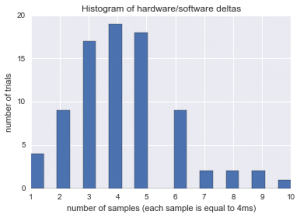```plt.plot(delay_time) plt.title('Delta over time') plt.xlabel('time (1 delta per trial)') plt.ylabel('delta (in samples)') plt.show()```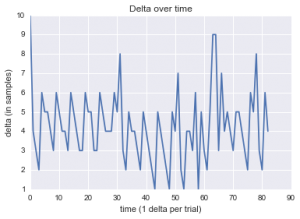What can we see in this data? Well first, in the top figure you can see that the distribution of deltas is (somewhat) normal distribution with a long tail to the right. On average, the delta is centered at 4 samples, which translates to 16ms. However, there is obviously some variance around that number, so we can’t just simply correct for that delay by subtracting it out. Another thing to notice is that there appears to be some regularity in the time course of the variance. The second graph above is the delta for each trial in the experiment. If you look closely, you can see that the delay seems to bounce back and forth between 3 and 6 samples, at least in the first 30 samples or so. This is a good sign! It means that there is possibly a way that we can predict the delay and correct for it.

# Final Thoughts and Future Experiments

Here, I presented some preliminary attempts at quantifying the delay between when we send a trigger from our OpenEXP software and when it actually arrives on the board. To test for the latency of this delay, we wired a photoresistor into the openbci board to calculate when stimuli are ACTUALLY displayed to the screen vs. when the trigger arrives in the data stream through the wireless connection. Counterintuitively, we observed that the photoresistor tag occured AFTER the software tag. This may be due to the an interaction with the refresh rate of the screen or possibly influenced by the timing of the trigger in our app. In a Part 2, we will investigate these possibilities and others. Nonetheless, this technique of calculating the delta between the photoresistor input from the screen and the software tag sent over the wireless connection could be very valuable for accurately tagging brain responses. Please help us solve this problem by commenting below or writing to me [email protected]

### One Commentjussijaatinen

Is it possible to send several different trigger codes to OpenBCI? With ERP studies most of times I need at least two, but usually more different triggers to be recorded to continuous raw eeg stream.